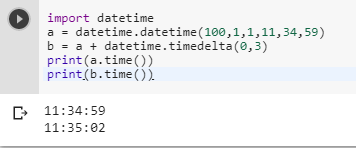2 views
in Python

Given a datetime.time value in Python, is there a standard way to add an integer number of seconds to it, so that 11:34:59 + 3 = 11:35:02, for example?

These obvious ideas don't work:

>>> datetime.time(11, 34, 59) + 3

TypeError: unsupported operand type(s) for +: 'datetime.time' and 'int'

>>> datetime.time(11, 34, 59) + datetime.timedelta(0, 3) TypeError: unsupported operand type(s) for +: 'datetime.time' and 'datetime.timedelta'

>>> datetime.time(11, 34, 59) + datetime.time(0, 0, 3)

TypeError: unsupported operand type(s) for +: 'datetime.time' and 'datetime.time'

In the end, I have written functions like this:

secs = timeval.hour * 3600 + timeval.minute * 60 + timeval.second

return datetime.time(secs // 3600, (secs % 3600) // 60, secs % 60)

I can't help thinking that I'm missing an easier way to do this though.

by (106k points)

Yes, in Python we have a datetime.time value and there is a standard way to add an integer number of seconds to it so that 11:34:59 + 3 = 11:35:02. Do do this you can use full datetime variables with timedelta, and by providing a dummy date then using the time to just get the time value.

Below is an example that shows how to do it:-

import datetime

a = datetime.datetime(100,1,1,11,34,59)

b = a + datetime.timedelta(0,3)

print(a.time())

print(b.time())

The results will be in the two values, with three seconds apart:Learn python with the help of this Python Course